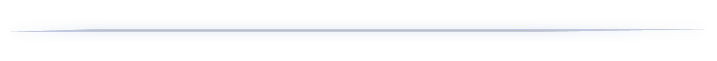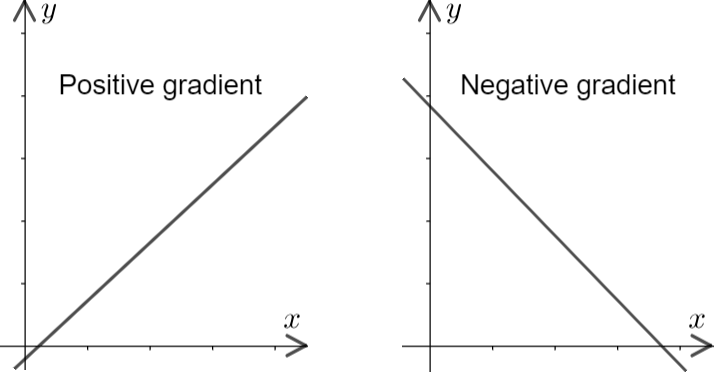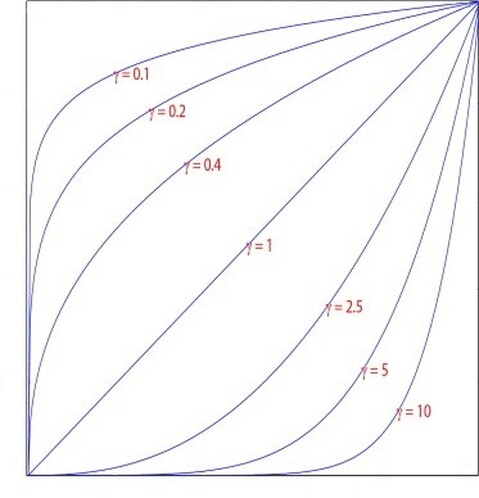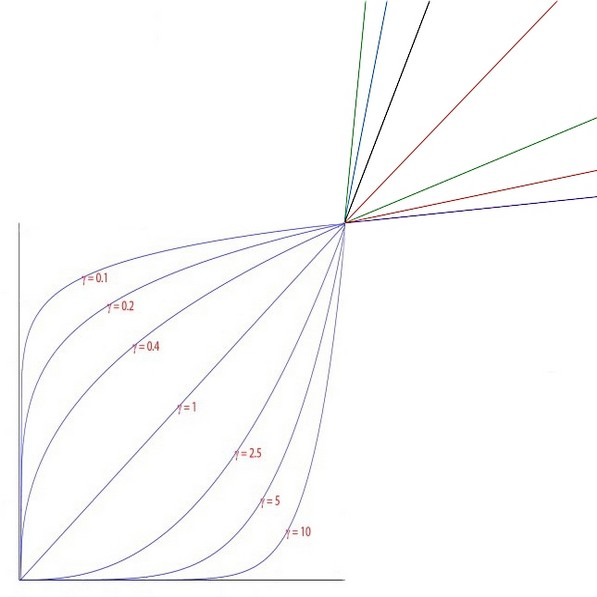# Analysing the Grade Node - Guillermo AlgoraGuillermo Algora
Go to content
FROM A MATHEMATICAL PERSPECTIVE

0. Introducing Equations of a Straight Line:

To begin, we must fully comprehend the concepts of the equation of a straight line, as it is key to the formulas in the Grade node.

The general equation of a straight line is:  y = mx + c

• y: is the ordinate or vertical plane.
• x: is the abscissa or horizontal plane.
• m: is the gradient or slope
• c: is the intersection of the ordinates or y-intercept (a constant value and the y value when x = 0).

•1. Analysing the Grade node from a mathematical perspective:

Fortunately, The Foundry has released the source code of some of the Nuke plugins (link), including the Grade node (link).
Concise information about how it works is to be found in the very first lines of the source code:

"Applies a linear ramp followed by a gamma function to each color channel.
A = multiply * (gain-lift)/(whitepoint-blackpoint)
B = offset + lift - A*blackpoint
output = pow(A*input + B, 1/gamma)
The reverse option is also provided [...]. This will do the opposite gamma correction followed by the opposite linear ramp."

The first line:
"Applies a linear ramp followed by a gamma function to each color channel"

Translates as:
The Grade node will apply an equation of straight line followed by a gamma function.

This is precisely what the last formula in the source code does:
output = pow(A*input + B, 1/gamma).

pow (base, exponent) is the C++ format to performing an exponential operation so that formula is in fact:
output = (A*input + B) ^ (1/gamma).

From here on, we will call that formula the final formula, as is the one in charge of producing the output (final result) of the node's operation.
To recap:

output  =   Applies a linear ramp followed by a gamma function to each color channel.
output  =                   (A*input + B)                          ^ (1/gamma)

Next, we see a rather simplified summary of the execution of the source code in regards to the application of such formulas (lines 79 to 158):
• Runs through the applicable channels → one at a time (e.g. first the red, then green, etc.) → pixel by pixel,
one at a time
(e.g. red channel, value of pixel at position
x, y
)
→ and
applies the formulas
→ then onto the next pixel until all resolution for that channel is completed → then continues with the next channel until all applicable channels are completed.

1.1 The final formula without the gamma function:

output = (A*input + B) (1/gamma)

The 'first part' of the final formula (the final formula without the gamma function) is an equation of a straight line:

output = A*input + Binput (x, the abscissa) is the input pixel value (before the transformation): e.g. red = 0.212.
output (y, the ordinate) is the resulting pixel value (after the transformation): e.g.  red = 0.4332.
value A is the slope.
and value B is the y-intercept.

In the following example, we will assume certain values for A and B to illustrate on the operation of the equation of a straight line:

input =  0.212
A = 1.1
B = 0.2
output = (A*input + B) → output = 1.1 * 0.212 + 0.2 → output = 0.4332

This rather simplistic example (the Grade node is rather simplistic in process, but not in its comprehensibility) shows the transformation of an input pixel value of 0.212 into an output pixel value of 0.4332 (for a given channel, e.g., red). This example could easily represent an operation in the Grade without gamma (since we have stripped that part of the equation for now).

Let's introduce
B next.

1.2 B:

B = offset + lift - A*blackpointThe formula that returns B is also an equation of a straight line. However, this equation of a straight line has a negative (or downward') slope. The negative sign in the slope means that any change in the value of x results in the opposite effect to the value of y:

Equation of a straight line with a positive slope:          Equation of a straight line with a negative slope:
- increment in x increment in y.                                                - increment in x reduction in y.
- reduction in x   reduction in y.                                                 - reduction in x   increment in y.This negative slope consolidates the function of the blackpoint knob. For in the formula of B, the blackpoint is x and any change in the value of x (blackpoint value) produces the opposite effect to the value of y (the B value). Increasing the blackpoint value reduces the output value to the point that when blackpoint value = input value output value = 0.

To illustrate, let us assume the following values:
input = 0.2
blackpoint = 0.2
All the other knobs at their default value

A = multiply * (gain-lift)/(whitepoint-blackpoint) A = 1 * (1-0)/(1-0.2) A = 1.25
B = offset + lift - A*blackpoint →  B = 0 - 1.25 * 0.2 →  B = - 0.25
output = input*A + B →  output = 0.2 * 1.25 + - 0.25 →  output = 0

Hence, b
ecause blackpoint drives the B value, and the B value (i.e. y-intercept) is the minimum output value in the final formula, the definition of blackpoint:

• Blackpoint: sets the black point (typically the darkest pixel).

Let's proceed to analyze offset and lift, which in the formula of B make the y-intercept. As y-intercept, they add a fixed value to y, in other words, to the B value.

• Offset: offsetting is to add a fixed value to the result.
• Lift: any pixels that are black are set to this color.

To illustrate this, let us build up from the previous example by adding values to offset and lift:
input = 0.2
blackpoint = 0.2
offset = 0.1
lift = 0.5
All the other knobs at their default value

A = multiply * (gain-lift)/(whitepoint-blackpoint) A = 1 * (1-0.5)/(1-0.2) A = 0.625
B = offset + lift - A*blackpoint →  B = 0.1 + 0.5 - 0.625 * 0.2 → B = 0.475
output = input*A + B →  output = 0.2 * 0.625 + 0.475 → output = 0.6

As we can see, a combined fixed value of 0.6 (offset: 0.1 + lift: 0.5) has been added to the output result (output value), because the value of B is the y-intercept of the final formula.

According to the above example, one might think that offset and lift serve the same function. However, that is not the case because lift is also present in the formula of A, where it finally sees its function redefined as the opposite of blackpoint.

Without further ado, let's analyze A.

1.3 A:

A is the slope in both the formula for B (B = offset + lift - A*blackpoint) and the final formula (output = A*input + B).

A = multiply * (gain-lift)/(whitepoint-blackpoint)

multiply multiplies the result of the right-hand side part of the formula (i.e. a multiplication to the slope value). This multiplication is effectively a multiplication to the output value but performed via the value of the slope (A value) so as not to affect the y-intercept value (B value), hence the definition:

• Multiply: multiplies the result of the Grade node by this factor.

The remaining part of the equation is the 'core' of the slope value:

(gain-lift)/(whitepoint-blackpoint)

The value for the slope is the result of a division between the two ends of the node's spectrum: gain, whitepoint; and lift, blackpoint. The result of a division between two numbers is in a sense a scale factor; in our case, it represents the change to the range of the image, the coefficient of input and output.

- Slope value = 1 no change (1:1 of input to output).
- Slope value > 1 increase of output.
- Slope value < 1 decrease of output.

While the above is the impact of the slope value as a whole, the function of each knob is defined by its position in the numerator (the top number of the fraction) or denominator (the bottom number of the fraction). While the commonality between gain, lift and whitepoint, blackpoint is defined by their shared position in the numerator or denominator field, their antagonism is defined by the mathematical operation of subtraction among them.

Because gain is in the numerator field: any change to its value produces the same effect on the slope value (A value) which carries the effect onto the output value. e.g. increase in gain value increase in slope value (A value) → increase in output value.

• Gain: any pixels that are white are set to this color.

To illustrate this, let us assume that:
input = 1
gain = 0.7
all other knobs are at their default value.

A = multiply * (gain-lift)/(whitepoint-blackpoint) → A = 1 * (0.7-0/1-0) →  A = 0.7
B = offset + lift - A*blackpoint →  B = 0 + 0 - 0.7 * 0 → B = 0
output = A * input + B →  output = 0.7 * 1 + 0 → output = 0.7

As we can see, the reduction of the gain value by 0.3 (from 1 to 0.7) produced (via the slope value) a reduction of 0.3 of the input value to the output value (from 1 to 0.7). That is: what was previously 1 (i.e. white) is now equal to the value of gain (0.7).

Because lift is also in the numerator field, the same 'dynamic' applies:

• Lift: any pixels that are black are set to this color.

To illustrate, let us assume that:
input = 0
lift = 0.3
all other knobs are at their default value.

A = multiply * (gain-lift)/(whitepoint-blackpoint) → A = 1 * (1-0.3/1-0) →  A = 0.7
B = offset + lift - A*blackpoint → B = 0 + 0.3 - 0.7 * 0 →  B = 0.3
output = A * input + B → output = 0.7 * 0 + 0.3 →  output = 0.3

As we can see, an input value of 0 has become equal to the value of lift.

On the other hand, whitepoint is in the denominator field, which means that any change to its value produces the opposite effect on the slope value (A value) and therefore on the output value. e.g. decrease in whitepoint value increase in slope value (A value) increase in output value.

That is, as we decrease the whitepoint value, the output value increases to the point that when whitepoint value = input value → output value = 1, which is precisely this knob's definition:

• Whitepoint: sets the white point (typically the lightest pixel). Any pixels of this value are set to 1. In other words, this color is turned into pure white.

To illustrate, let us assume that:
input = 0.7
whitepoint = 0.7
all other knobs are at their default value.

A = multiply * (gain-lift)/(whitepoint-blackpoint) → A = 1 * (1-0/0.7-0) →  A = 1,4285
B = offset + lift - A*blackpoint → B = 0 + 0 - 0.7 * 0 →  B = 0
output = A * input + B → output =1,4285 * 0.7 + 0 →  output = 1

As blackpoint is also in the denominator field, any change to its value produces the opposite effect on the slope value:

• Blackpoint: sets the black point (typically the darkest pixel).

To illustrate this, let us assume that:
input = 0.3
blackpoint = 0.3
all other knobs are at their default value.

A = multiply * (gain-lift)/(whitepoint-blackpoint) →  A = 1 * (1-0/1-0.3) →  A = 3,3333
B = offset + lift - A*blackpoint → B = 0 + 0 – 3,3333*0.3 →  B = -1
output = A * input + B → output =3,3333 * 0.3 - 1 →  output = 0

As we have seen, because of their shared position in the fields of the division and in regards to the slope value: lift has the same dynamic as gain and blackpoint as whitepoint, however, lift and blackpoint are (despite the two other) present in the formula of B where they ultimately see their function defined.

Contrary to certain misconceptions, multiply and gain do not have the same function. However, they are the same if the rest of the other knobs are at their default value (excluding gamma, as it being outside the formula of the equation of a straight line does not apply as it is ).

1.4 The gamma function:

The complete final formula consists of the equation of a straight line followed by a gamma function. The gamma function is the part in which the result from the equation of a straight line (the base) is raised to 1/gamma (the exponent):

output = (A*input + B) (1/gamma)

The function of the gamma knob is carried out via the gamma function as a result of the following intrinsic property of the exponential operations: the strength of the multiplicative effect is maximal when the value of the base is 0.5 and decreases as the value of the base approaches either 0 or 1.

Let us illustrate in the following example:
0.15 2 = 0.0225
0.5 2 = 0.25
0.85 2 = 0.7225

The effect has been maximal when the base is 0.5 (0.5 - 0.25 = 0.25 difference), but as the base is close to 0 (0.15) the effect has been reduced (0.15 - 0.0225 = 0.1275 difference) as well as when the base is close to 1 (0.85) (0.85 - 0.7225 = 0.1275 difference).

• Gamma: applies a constant gamma value to the result of the Grade node. This lightens or darkens the midtones.However, as shown in the example above, raising a base value of less than 1 to a higher exponent value produces, rather than an increase, a decrease in the output value. Hence, to avoid it exists the division of 1 by the value of gamma in the formula, to invert the value of gamma (e.g. 1/0.75 = 1,333) so that any change in the gamma value produces an effect in the same 'direction' on the output value.

To illustrate this, the following chart shows a comparison:
 Gamma value: 2 Gamma value with 1/gamma → 1/2 = 0.5 0.15 2 = 0.0225    0.15 (1/2) = 0.3872 0.5 2 = 0.25 0.5 (1/2) = 0.7071 0.85 2 = 0.7225 0.85 (1/2) = 0.9219

1.5 The hidden 'side' of the gamma function:

So far, until now we have worked with the information easily accessible in the first lines of source code, however, the gamma function has some 'additional' parameters established within the code itself. These parameters (lines: 128 to 153) establish that starting from the result of the equation of a straight line (i.e. the value of the base) when performing the gamma function:

• If the value of the base is less than 0: the output will be the value of the base, in other words, the gamma function is discarded. This is because any value less than 0 is considered to be outside the range of action of gamma.

• If the value of the base is greater than 1, the gamma function is replaced by: (base value -1) * (1/gamma value) + 1, which is in fact another equation of a straight line (i.e. y = m*x + c). This means that from a value of 1, the gamma knob continues the trend of the gamma function curve in a linear progression.Back to content